﻿ Q235钢板对高速弹的抗侵彻特性研究
 舰船科学技术2017, Vol. 39Issue (2): 52-54PDF
Q235钢板对高速弹的抗侵彻特性研究

Ballistic performance of Q235 steel plate subjected to impact by middle and high velocity projectiles
ZHANG Yuan-hao, CHEN Chang-hai, ZHU Xi
Department of Naval Architecture Engineering, Naval University of Engineering, Wuhan 430033, China
Abstract: In order to investigate the ballistic performance and failure modes of metal plates subjected to impact by typical defense target projectiles, carried out a series of ballistic experiment, and combine ANSYS/LS-DYNA, investigated the influence of the ballistic performance by projectiles initial velocity、incidence angle of target, contrast and analysis the failure modes in different conditions. The results indicated that, the higher the initial velocity、the bigger the incidence angle、the bigger the thickness of metal plates, the better ballistic performance of metal plates.
Key words: impact     projectile     target
0 引 言

1 实验设计及结果

45# 钢及 Q235 钢的材料参数如表 1 所示。表 1 材料性能参数 Tab.1 Material properties表 2 3.30 g 立方体弹侵彻 Q235 钢板弹道实验结果 Tab.2 The results of metal plates subjected to impact by 3.30 g and 2.20 g target projectiles
2 有限元仿真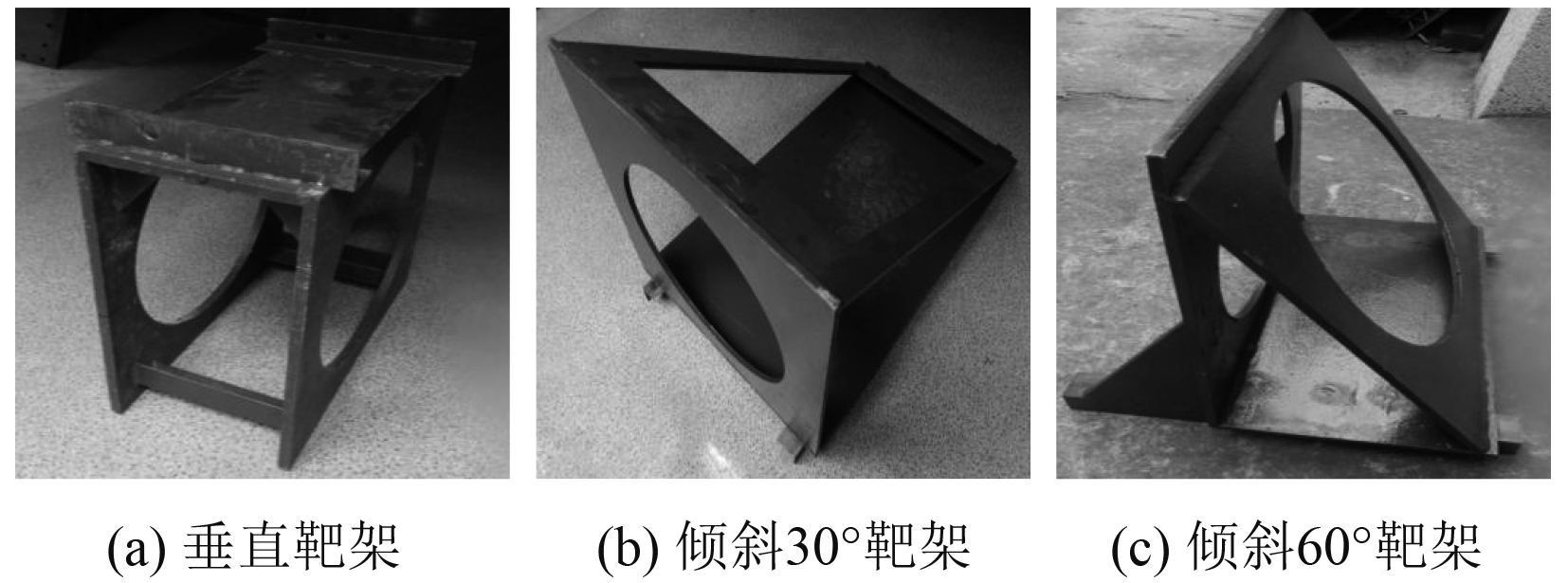图 1 不同倾斜角度的靶架 Fig. 1 Targets in different inclination angle
 ${\sigma _{d}} = \left( {{\sigma _0} + \frac{{E{E_{{h}}}}}{{E - {E_{{h}}}}}{\varepsilon _{{p}}}} \right)\left[ {1 + {{\left( {\frac{{\dot \varepsilon }}{D}} \right)}^{1/{{n}}}}} \right]\text{，}$ (1)

 ${\sigma _y} = (A + B{\varepsilon ^p}^n)\left[ {1 + c\ln \frac{{{\varepsilon ^p}}}{{{\varepsilon _0}}}} \right]\left[ {1 - {{\left( {\frac{{T - {T_0}}}{{{T_m} - {T_0}}}} \right)}^m}} \right]\text{，}$ (2)

 ${\varepsilon _{\rm{f}}} \!\!=\!\! \left[\! {{D_1} \!\!+\!\! {D_2}\exp \left( \!{{D_3}\frac{{{\sigma _{\rm{h}}}}}{{{\sigma _{{\rm{eff}}}}}}} \right)} \!\right]\left( \!\!{1 \!\!+\!\! {D_4}\frac{{{\varepsilon ^p}}}{{{{\dot \varepsilon }_{\rm{0}}}}}} \!\!\! \right)\left[ \!\!{1 \!\!+\!\! {D_5}\left( {\frac{{T\!\! -\!\! {T_0}}}{{{T_m} \!\!-\!\! {T_0}}}} \right)} \!\right]\text{。}$

3 实验及计算结果分析 3.1 靶板侵彻过程及破坏模式分析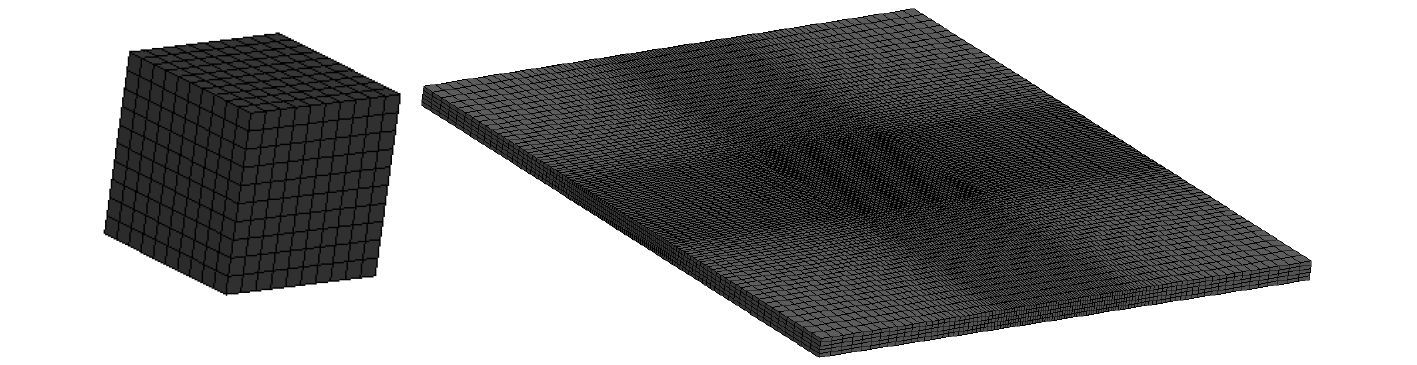图 2 立方体弹、长方体弹及钢板模型示意图 Fig. 2 Sketch of finite element model for cube projectile,cuboid projectile and steel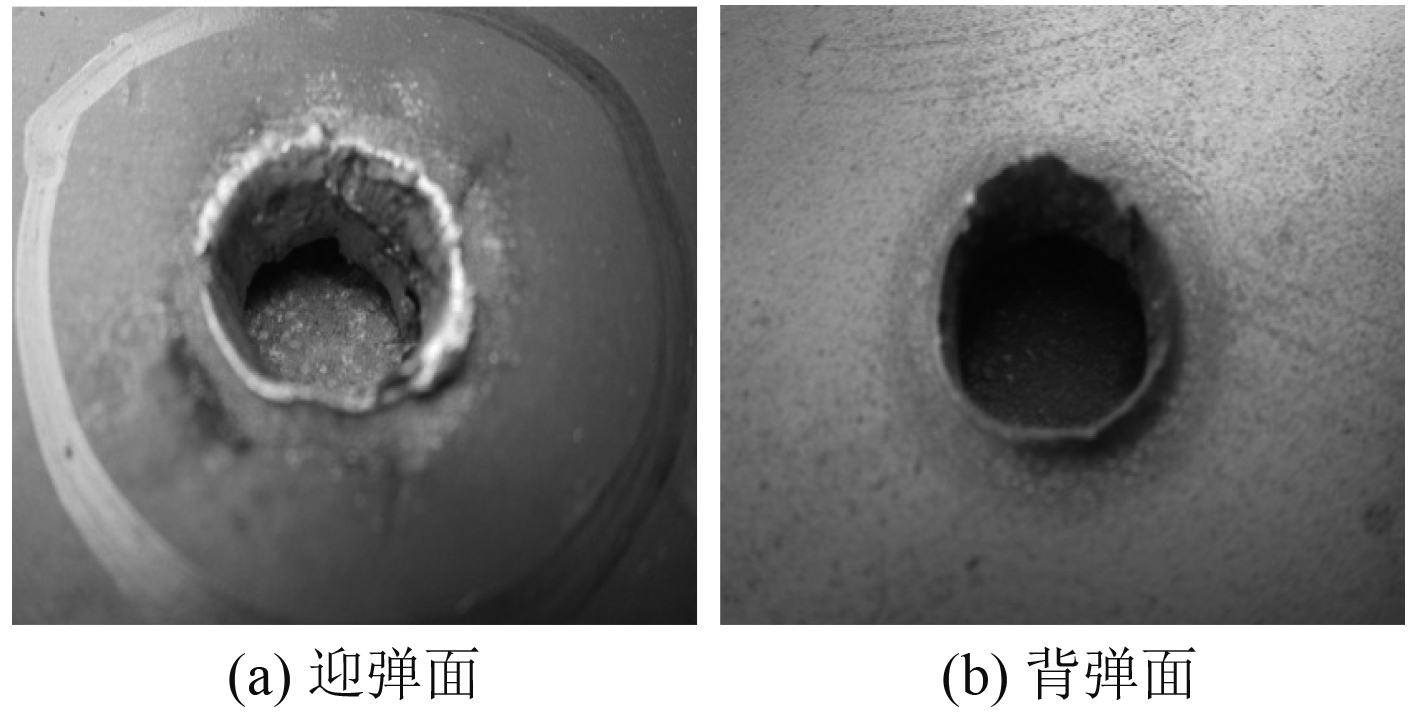图 3 工况 2 靶板的破坏形貌 Fig. 3 Failure mode 2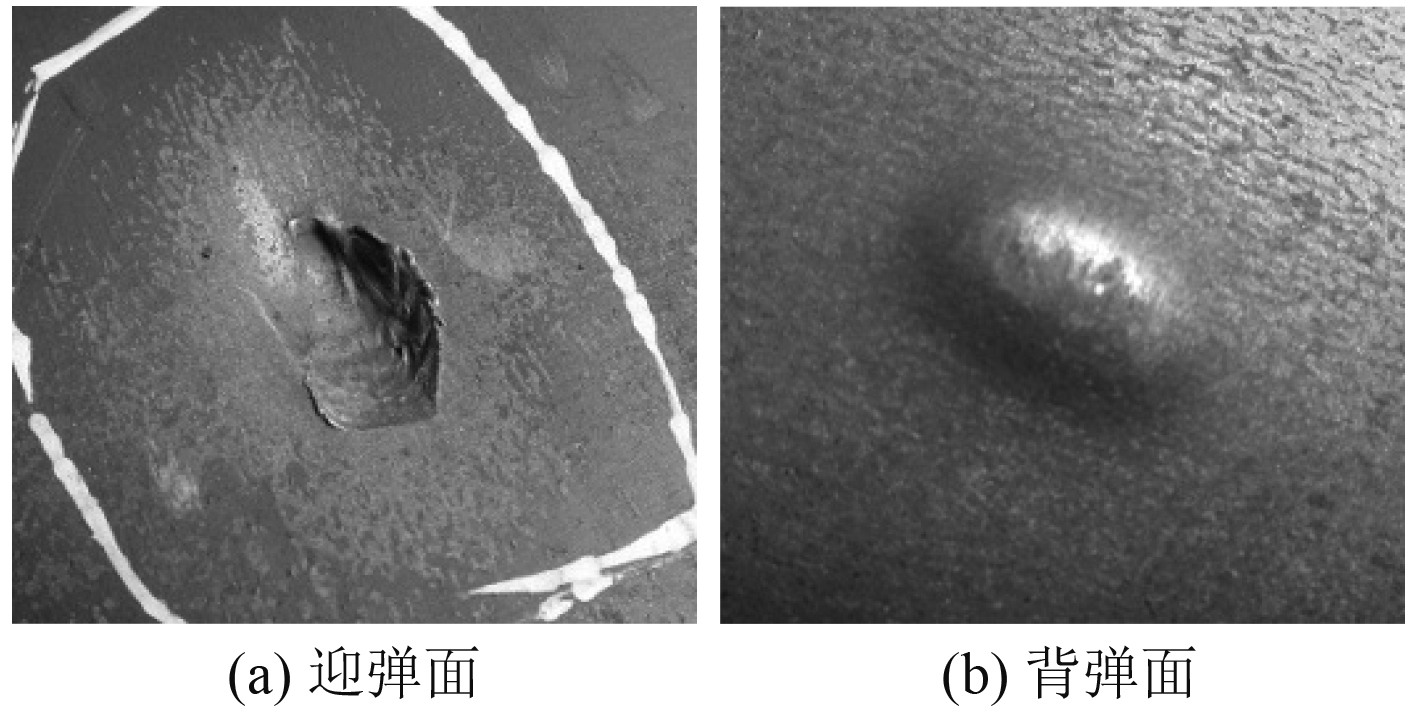图 4 工况 5 靶板的破坏形貌 Fig. 4 Failure mode 5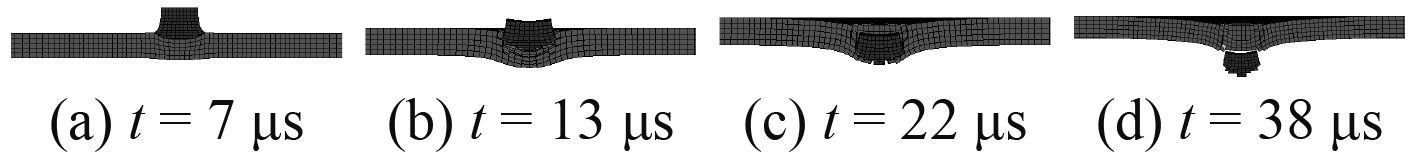图 5 工况 2 侵彻过程仿真 Fig. 5 Penetration process emulation 2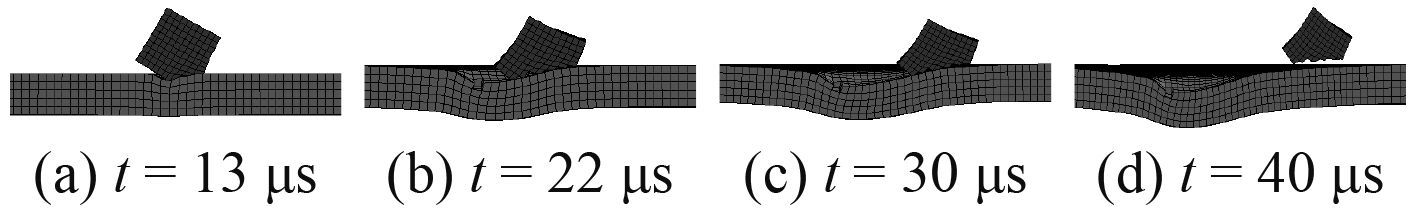图 6 工况 5 侵彻过程仿真 Fig. 6 Penetration process emulation 5
3.2 抗弹性能影响因素分析 3.2.1 靶板厚度影响因素分析

3.2.2 弹丸初速影响因素分析

3.2.3 弹体入射角影响因素分析

4 结 语

  潘建华, 文鹤鸣. 平头弹丸撞击下延性金属靶板的破坏模式[J]. 高压物理学报, 2007, 21 (2): 157–164.  刘文韬, 张数道, 李恩征. 金属材料侵彻的理论分析和数值模拟研究[R]. 中国国防科学技术报告, GF-A-0061577G, 2004.  朱学旺, 黄寅生, 李永池. 贯穿有限厚金属靶板的一种模型[J]. 弹道学报, 2001, 13 (2): 1–6.  张国伟. 爆炸作用原理[M]. 北京: 国防工业出版社, 2006.  蒋志刚, 曾首义, 周建平. 金属薄靶板冲塞破坏最小穿透能量分析[J]. 工程力学, 2004, 21 (5): 203–208.  CHEN X W, LI Q M. Shear plugging and perforation of ductile circular plates struck by a blunt projectile[J]. International Journal of Impact Engineering, 2003, 28 (5): 513–536. DOI: 10.1016/S0734-743X(02)00077-5  王儒策, 赵国志. 弹丸终点效应[M]. 北京: 北京理工大学出版社, 1993.  马晓青, 韩峰. 高速碰撞动力学[M]. 北京: 国防工业出版社, 1998.  TENG X, DEY S, BORVIK T, et al. Protection performance of double-layered metal shields against projectile impact[J]. Journal of Mechanics of Materials and Structures, 2007, 2 (7): 1309–1330. DOI: 10.2140/jomms  TENG X, WIERZBICKI T, HUANG M. Ballistic resistance of double-layered armor plates[J]. International Journal of Impact Engineering, 2008, 35 (8): 885–894. DOI: 10.1016/j.ijimpeng.2008.01.006